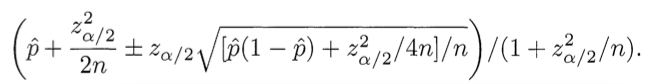# Agent ranking formula

(Originally published on  Evan Millers’ Blog)

PROBLEM: You are a web programmer. You have users. Your users rate stuff on your site. You want to put the highest-rated stuff at the top and lowest-rated at the bottom. You need some sort of "score" to sort by.

WRONG SOLUTION #1: Score = (Positive ratings) - (Negative ratings)

Why it is wrong: Suppose one item has 600 positive ratings and 400 negative ratings: 60% positive. Suppose item two has 5,500 positive ratings and 4,500 negative ratings: 55% positive. This algorithm puts item two (score = 1000, but only 55% positive) above item one (score = 200, and 60% positive). WRONG.

WRONG SOLUTION #2: Score = Average rating = (Positive ratings) / (Total ratings)

Why it is wrong: Average rating works fine if you always have a ton of ratings, but suppose item 1 has 2 positive ratings and 0 negative ratings. Suppose item 2 has 100 positive ratings and 1 negative rating. This algorithm puts item two (tons of positive ratings) below item one (very few positive ratings). WRONG.

CORRECT SOLUTION: Score = Lower bound of Wilson score confidence interval for a Bernoulli parameter

Say what: We need to balance the proportion of positive ratings with the uncertainty of a small number of observations. Fortunately, the math for this was worked out in 1927 by Edwin B. Wilson. What we want to ask is: Given the ratings I have, there is a 95% chance that the "real" fraction of positive ratings is at least what? Wilson gives the answer. Considering only positive and negative ratings (i.e. not a 5-star scale), the lower bound on the proportion of positive ratings is given by:(Use minus where it says plus/minus to calculate the lower bound.) Here   is the observed fraction of positive ratings, zα/2 is the (1-α/2) quantile of the standard normal distribution, and n is the total number of ratings. The same formula implemented in Ruby:

`require 'statistics2' def ci_lower_bound(pos, n, confidence)      if n == 0          return 0      end      z = Statistics2.pnormaldist(1-(1-confidence)/2)      phat = 1.0*pos/n      (phat + z*z/(2*n) - z * Math.sqrt((phat*(1-phat)+z*z/(4*n))/n))/(1+z*z/n) end`

`pos`  is the number of positive ratings, `n`  is the total number of ratings, and `confidence`  refers to the statistical confidence level: pick 0.95 to have a 95% chance that your lower bound is correct, 0.975 to have a 97.5% chance, etc. The z-score in this function never changes, so if you don't have a statistics package handy or if performance is an issue you can always hard-code a value here for `z` . (Use 1.96 for a confidence level of 0.95.)

#### REFERENCES

Binomial proportion confidence interval (Wikipedia)

Agresti, Alan and Brent A. Coull (1998), "Approximate is Better than 'Exact' for Interval Estimation of Binomial Proportions,"  The American Statistician, 52, 119-126.

Wilson, E. B. (1927), "Probable Inference, the Law of Succession, and Statistical Inference,"  Journal of the American Statistical Association, 22, 209-212.